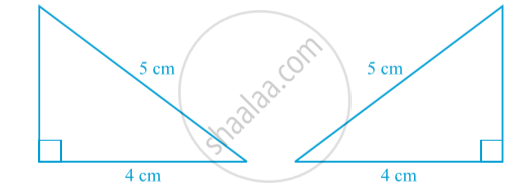# Some More Criteria for Congruence of Triangles

#### theorem

Theorem (SSS congruence rule) :  If three sides of one triangle are equal to the three sides of another triangle, then the two triangles are congruent.
Construct two right angled triangles with hypotenuse equal to 5 cm and one side equal to 4 cm each in following fig.The two triangles cover each other completely and so they are congruent.
Note that, the right angle is not the included angle in this case.

Theorem (RHS congruence rule) :  If in two right triangles the hypotenuse and one side of one triangle are equal to the hypotenuse and one side of the other triangle, then the two triangles are congruent.
Note that RHS stands for Right angle - Hypotenuse - Side.

If you would like to contribute notes or other learning material, please submit them using the button below.

### Shaalaa.com

Theorem : SSS (Side Side Side) [00:03:11]
S
0%